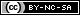# NGS Analysis with R

### Using R packages

1. ShortRead - Quality assesment of the reads, finding duplicates, trimming, string pattern searches 
2. Biostrings - Reading sequences in R 
3. BSGenomes - Reading in complete genomes and BioC annotation data 
4. DEGSeq - Identify differentially expressed genes from RNA-Seq data. 
5. IRanges - infrastructure for positional data.
6. biomaRt - interface to BioMart annotations.
7. rtracklayer - interface to online and other genome browsers.
8. chipseq & ChIPpeakAnno - Chip-Seq

### Parsing Output with R

R
`library(ShortRead)`

`alignedReads <- readAligned("./", pattern="output.bowtie", type="Bowtie")`
`   # Reads in alignment coordinates as a ShortRead AlignedRead object`
`alignedReads <- readAligned("./", pattern="output.bowtie", type="SOAP")`

### RNA-Seq Analysis

```########################## ## Import Aligned Reads ## ########################## library(GenomicRanges); library(Rsamtools); library(leeBamViews) # Load required libraries. testFile <- system.file("bam", "isowt5_13e.bam", package = "leeBamViews") aligns <- readBamGappedAlignments(testFile) # Imports a BAM alignment file (here yeast example) and stores it as a GappedAlignments object.```
`rname(aligns) <- sub("^Sc", "", rname(aligns)); ``rname(aligns) <- sub("13", "XIII", rname(aligns))`` # Required for data consistency.`
`alignscan <- scanBam(testFile); names(alignscan[]) # Imports BAM data into a nested list object. `######################################################

## Organize Annotation Data in a GRangesList Object ##

######################################################
library(GenomicFeatures)
txdb <- makeTranscriptDbFromUCSC(genome="sacCer2", tablename="sgdGene") # Creates a TranscriptDb object from transcript annotations available at the UCSC Genome Browser.
exonRanges <- exonsBy(txdb, "tx") # Stores exon data as a GRangesList object.```####################################################### ## Create Annotation Objects from Custom Data Frames ## #######################################################```
```transcripts <- data.frame(tx_id=1:3, tx_chrom="chr1", tx_strand=c("-", "+", "+"), tx_start=c(1, 2001, 2001), tx_end=c(999, 2199, 2199)) splicings <- data.frame(tx_id=c(1L, 2L, 2L, 2L, 3L, 3L), exon_rank=c(1, 1, 2, 3, 1, 2), exon_start=c(1, 2001, 2101, 2131, 2001, 2131), exon_end=c(999, 2085, 2144, 2199, 2085, 2199), cds_start=c(1, 2022, 2101, 2131, NA, NA), cds_end=c(999, 2085, 2144, 2193, NA, NA)) myTxdb <- makeTranscriptDb(transcripts, splicings) # Example for creating TranscriptDb object from two data frames. exonsBy(myTxdb, "tx") ````# Returns exon data as GRangesList object.`

```################################################### ## Counting Reads that Overlap Annotation Ranges ## ###################################################```
```counts <- countOverlaps(exonRanges, aligns) # Counts matching reads per transcript. numBases <- sum(width(exonRanges)) # Number of bases per exonRanges element. geneLengthsInKB <- (numBases/1000) # Conversion to kbp. millionsMapped <- sum(counts)/1e+06 # Factor for converting to million of mapped reads. rpm <- counts/millionsMapped # ````RPK: reads per kilobase of exon model.`
`rpkm <- rpm/geneLengthsInKB # RPKM: reads per kilobase of exon model per million mapped reads. Note: the results are stored in a named vector that matches the index of the initial GRangesList object!!!`

sortedRPKM <- sort(rpkm); highScoreGenes <- tail(sortedRPKM)
txs <- transcripts(txdb, vals = list(tx_id = names(highScoreGenes)), columns = c("tx_id", "gene_id"))

systNames <- as.vector(unlist(elementMetadata(txs)["gene_id"])) # Example for returning the six most highly expressed transcripts.

```################################################################### ## Counting Reads for Non-Annotation Ranges (Intergenic Regions) ## ###################################################################```
```filtData <- subsetByOverlaps(aligns, exonRanges) # Returns all reads that align in non-exon ranges. This includes introns which are rare in this example. filtData2 <- subsetByOverlaps(aligns, transcriptsBy(txdb, "gene")) ````# Returns all reads that align in intergenic regions (excludes introns).`
```cov <- coverage(filtData) # Calculates coverage for non-exon ranges. cov <- cov # Subsetting of chromosome 13 because data for the other chromosomes is missing in this example. islands <- slice(cov, lower = 1) # Filters for areas with a continuous read coverage of >=1. transcribedRegions <- islands[width(islands) > 1000] # Returns only those transcribed regions that are at least 1000 bp long.```This work is licensed under a Creative Commons Attribution-NonCommercial-ShareAlike 4.0 International License. Permissions beyond the scope of this license may be available at Attribution.